Mobile QR Code1. (School of Electronic Engineering, Kumoh National Institute of Technology, Gumi, Gyungbuk, Korea)

Pipelined SAR ADC, redundancy generation, dynamic amplifier, input range calibration, digital error correction

## I. INTRODUCTION

Recently, as the role of digital signal processing (DSP) increases, the importance of analog-to-digital converters (ADCs) for converting analog signals existing in nature into digital signals is increasing. Furthermore, the resolution of ADCs required for applications such as mobile systems and internet of things (IoT) is continuously increasing, and the demands for smaller area and low power consumption are steadily increasing. Thus, ADCs with a pipelined successive approximation register (SAR) architecture were used to implement conversion rates of tens of MS/s and dynamic range more than about 60dB while consuming a low power (1,2). A SAR ADC, which replaces a flash ADC used for a sub-ADC in a pipelined SAR ADC, can minimize the power consumption of analog blocks by using a capacitor-based digital-to-analog converter (DAC). Furthermore, dynamic amplifiers have been recently used to reduce the static current consumption of residual amplifiers used in pipelined ADCs (3).

However, these design trends can lead to the following issues. First, the charge sharing generated by the capacitor-based DAC used for the sub-ADC affects the residue voltage value of the coarse ADC and causes a change in the input range of the next-stage fine ADC. Next, dynamic amplifiers typically do not use a feedback structure to reduce power consumption. In this case, a residue amplifier that uses a dynamic amplifier may have variations in voltage gain and poor linearity. The two issues mentioned above can degrade overall performance, including the linearity of pipelined SAR ADCs.

In this paper, a 1-bit redundancy generation in a coarse ADC, an input range calibration for a fine ADC, and a linearity-improved dynamic amplifier for a residue amplifier are proposed for a 100-MS/s 10-bit pipelined SAR ADC using a 1.2-V supply voltage (4). Three proposed schemes improve the performance of the pipelined SAR ADC while reducing power consumption. Section II describes the architecture of the proposed pipelined SAR ADC. In addition, the proposed coarse ADC, fine ADC, and residue amplifier are introduced in detail. The implementation and measured results of the proposed pipelined SAR ADC are discussed in Section III. Finally, Section IV concludes this paper.

Fig. 1. Proposed pipelined SAR ADC (a) block diagram, (b) timing diagram.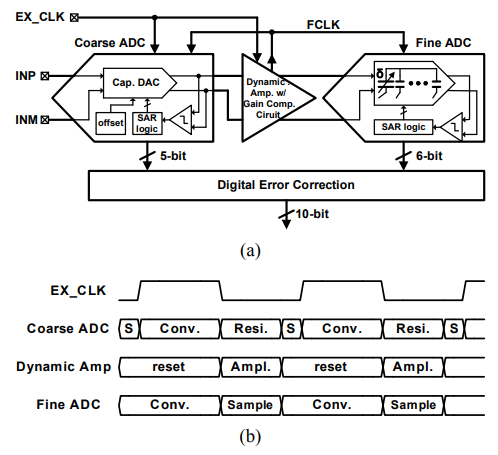## II. PROPOSED PIPELINED SAR ADC

Fig. 2. Proposed 5-bit coarse SAR ADC (a) block diagram, (b) timing diagram.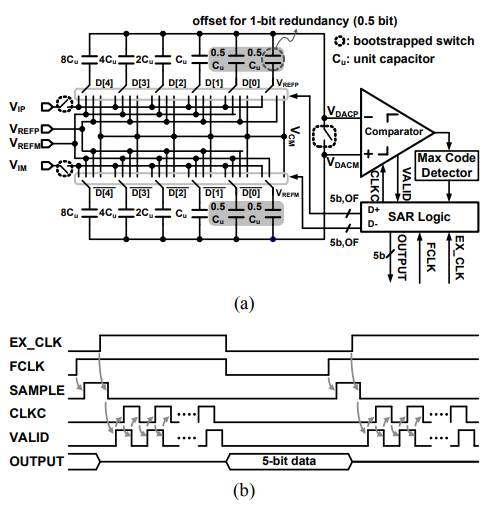### 1. 5-bit Coarse SAR ADC with 1-bit Redundancy Generation using Capacitor-based DAC

The 5-bit coarse SAR ADC in the proposed pipelined SAR ADC generates the residue voltage for 1-bit redundancy in to adopt the digital error correction used in conventional pipelined ADCs. As shown in Fig. 2(a), it consists of a 5-bit capacitor-based DAC, a comparator, and a SAR logic with a maximum code detector to generate 4-bit digital output data and 1-bit redundancy. The 5-bit capacitor-based DAC for the coarse SAR ADC has a V$_{\mathrm{CM}}$-based capacitor architecture consisting of binary weighted capacitors (5). In general, the capacitor-based DAC of a SAR ADC has two 1·C$_{U}$s for lest significant bit (LSB) conversion. One of two 1·C$_{U}$s is switched by using the determined LSB code and the other maintains a connection to AC ground (V$_{CM}$). However, in this work, the final 1·C$_{U}$ of the 5-bit capacitor-based DAC is divided into two 0.5·C$_{U}$s for the operation of the residue generation required in the conventional pipelined ADC. One 0.5·C$_{U}$ should be switched by using the determined LSB code D, then the 5-bit capacitor-based DAC outputs a residue voltage after the 5-bit conversion of the coarse ADC. Therefore, the maximum residue voltage is determined as the analog voltage for 0.5 LSB. In addition, another 0.5·C$_{U}$ is used to generate 1-bit redundancy for the digital error correction. The 5-bit capacitor-based DAC is also used as the sampling circuit, the first circuit in the proposed pipelined SAR ADC. Therefore, to reduce the thermal voltage generated by the sampling circuit, the 0.5·C$_{U}$ is designed to be 14.5 fF larger than the minimum value that can be implemented using metal-oxide-metal (MOM) capacitors. The comparator includes a meta-stability detector for stable asynchronous SAR operation (6). The SAR logic is controlled by the results of the comparator and max code detector for the digital error correction of the pipelined SAR ADC.

Fig. 3. Operation of capacitor-based DAC for offset voltage application.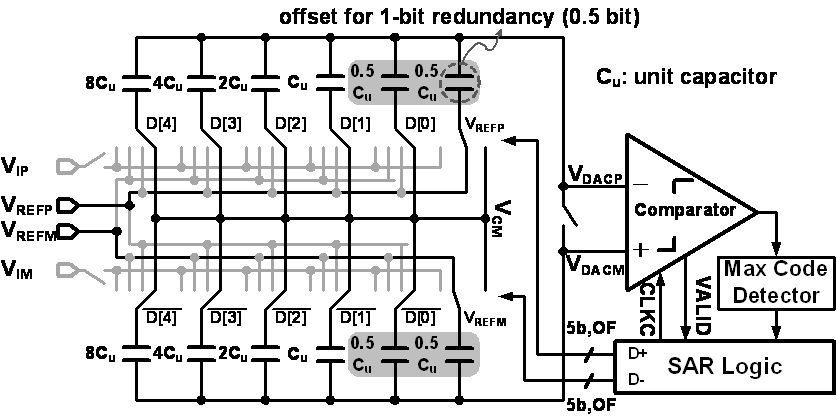Fig. 2(b) shows the timing diagram of the 5-bit coarse SAR ADC. The coarse SAR ADC samples the analog input signal from the rising edge of FLCK to the rising edge of EX_CLK, for the first conversion process. The nodes of all the top plates of the capacitors of the capacitor-based DAC, V$_{DACP}$ and V$_{DACM}$, are shorted each other and determined by the common mode voltage V$_{CM}$. All the bottom plates of the capacitors of the capacitor-based DAC are connected to the differential analog input signals of V$_{IP}$ and V$_{IM}$. In the second conversion process, V$_{CM}$ is applied to all the bottom plates of the capacitors in the capacitor-based DAC. In this case, both nodes V$_{DACP}$ and V$_{DACM}$ are disconnected and remain in a high impedance state. Through the first and second conversion processes, V$_{DACP}$ and V$_{DACM}$ are generated by the relationship of Eq. (1)which determines the most significant bit (MSB) code in the conventional SAR ADC.

Fig. 4. Transfer curve of residue voltage for 5-bit coarse SAR ADC (a) transfer curve for 5 bits, (b) transfer curve with added offset voltage, (c) proposed transfer curve for 4.5 bits.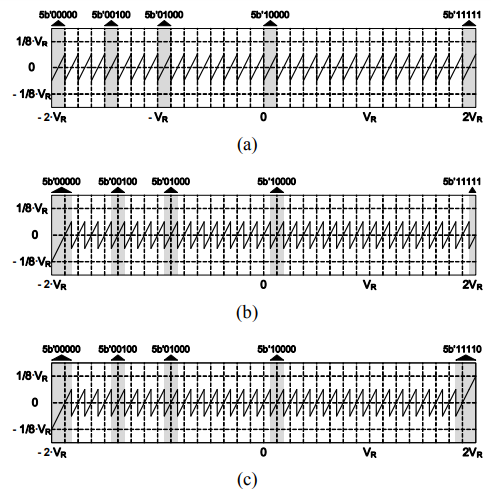##### (1)
V$_{\mathrm{DAC\_DIFF}}$ = V$_{\mathrm{DACM}}$ ${-}$ V$_{\mathrm{DACP}}$ = V$_{\mathrm{IP}}$ ${-}$ V$_{\mathrm{IM}}$

However, a certain offset voltage should be added to Eq. (1)to implement the SAR ADC with 1-bit redundancy. The offset voltage is added using 0.5·C$_{U}$ between the sample and data conversion processes. To perform the addition of the offset voltage, two 0.5·C$_{U}$s are connected to V$_{REFP}$ and V$_{REFM}$, respectively, as shown in Fig. 3. In this case, V$_{DAC\_DIFF}$, which is the differential voltage of the capacitor-based DAC and is determined by the difference between V$_{REFM}$ and V$_{REFP}$, is defined by Eq. (2), where V$_{R}$ is equal to (V$_{REFP}$ - V$_{REFM}$)/2.

##### (2)
V$_{DAC\_DIFF}$ = V$_{IP}$ ${-}$ V$_{IM}$ ${-}$ 1/16 · V$_{R}$

After the process of adding this offset voltage, the data conversion process is performed through the basic operation of the asynchronous SAR ADC. The proposed 5-bit coarse SAR ADC with 1-bit redundancy determines the MSB code, D, from Eq. (2). Applying the determined D to the 0.5·C$_{U}$ of the 5-bit capacitor-based DAC, the capacitor-based DAC generates the residue voltage needed for the sub-ADC of the pipelined ADC. The differential residue voltage is determined by Eq. (3).

##### (3)
$V_{D A C\_ D I F F}=V_{I P}-V_{I M}-\frac{1}{2^{4}} \cdot V_{R}+V_{R} \cdot\left\{\frac{(-1)^{D}}{2^{0}}+\cdots+\frac{(-1)^{D}}{2^{4}}\right\}$

Fig. 4 shows the transfer curve of the residue voltage to generate effective 4.5 bits by having 1-bit redundancy according to Eq. (3). Fig. 4(a) shows the typical transfer curve of the residue voltage for five bits. The additional switch of the 0.5·C$_{U}$ shifts this transfer curve by the offset voltage of 1/16·V$_{R}$, as shown in Fig. 4(b). This offset voltage corresponds to 0.5 LSB of the 5-bit coarse SAR ADC. Finally, the transfer curve of the residue voltage for the effective 4.5 bits is implemented using the maximum code detector, as shown in Fig. 4(c). It is possible to design using only adder operations for the digital error correction in the conventional pipelined ADC because the maximum code detector changes 5b’11111 to 5b’11110. In this case, the capacitor-based DAC generates a maximum residue voltage of 1 LSB. The 1-bit redundancy is generated through the process described above.

The behavioral simulations based on the block diagram in Fig. 1 (a) were performed to verify the performance improvement due to the 1-bit redundancy generation used in the proposed 5-bit coarse SAR ADC. Fig. 5 shows the stochastic simulation results of the performance of the ADC when the capacitor mismatch in the 5-bit coarse SAR ADC and the voltage gain error in the residue amplifier occur randomly between 1% and 3%. These mismatch values were determined by considering the input-dependent voltage gain variation of the dynamic amplifier used as the residue amplifier and the relative mismatch between the capacitors used in the 5-bit SAR ADC. In these simulations, the pipelined SAR ADC for the behavioral simulations without the 1-bit redundancy generation consists of a 4-bit coarse ADC, a residue amplifier, and a 6-bit fine ADC. Furthermore, it is assumed that there is no error in the fine ADC. Fig. 5(a)-(c) show the signal to noise and distortion ratio (SNDR), differential nonlinearity (DNL), and integral nonlinearity (INL) among the various performances of the 10-bit pipelined SAR ADC. The 1-bit redundancy generation for the 5-bit coarse SAR ADC improves all three performances. In particular, it reduces the DNL and INL performances of the 10-bit pipelined SAR ADC to less than ${\pm}$ 1.0 LSB when both the capacitor mismatches in the 5-bit coarse SAR ADC and the voltage gain error in the residue amplifier occur randomly within 1%.

Fig. 5. Behavioral simulation results with and without 1-bit redundancy generation (a) SNDR, (b) DNL, (c) INL.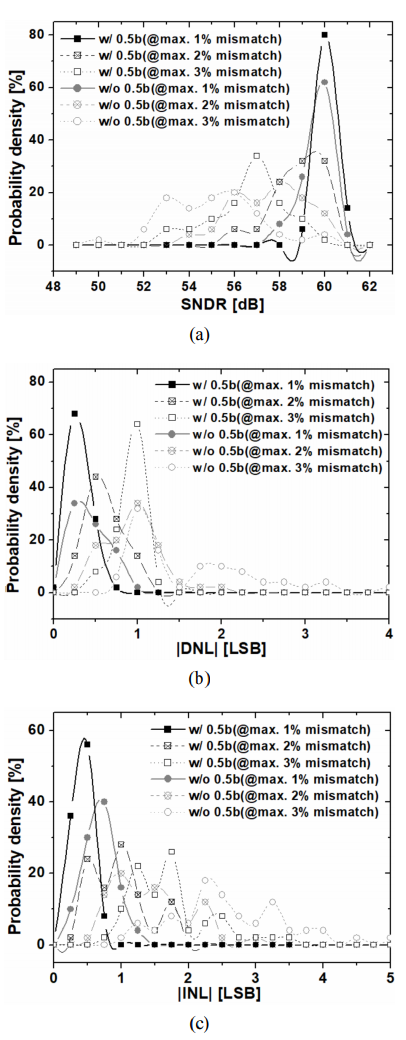### 2. Linearity-improved Dynamic Amplifier

In order to reduce the power consumption of the pipelined SAR ADC proposed in this work, the dynamic amplifier shown in Fig. 6 is used instead of a static amplifier. This dynamic amplifier has an open loop architecture to improve the settling time (3). In Fig. 6(a), when CLK goes low, the voltage values of V$_{1}$ and V$_{2}$ nodes are sampled at the V$_{OUTM}$ and V$_{OUTP}$ nodes, respectively. The pre-charge phase shown in Fig. 6(b) also starts. The active loads, MP0 and MP1, are turned on and an enable switch, MN0, is turned off. Therefore, V$_{OUTP}$ and V$_{OUTM}$ are charged to the supply voltage of V$_{DD}$. When CLK is high, MP0 and MP1 are turned off and MN0 is turned on. Also, the V$_{1}$ and V$_{OUTM}$ nodes and the V$_{2}$ and V$_{OUTP}$ nodes are connected to each other. In this mode, the amplification process of the dynamic amplifier is performed while V$_{1}$ and V$_{2}$ nodes are discharged to the ground voltage of V$_{SS}$ depending on the input voltages V$_{IP}$ and V$_{IM}$ of the dynamic amplifier. The common mode voltage detector generates a flag while discharging V$_{1}$ and V$_{2}$ nodes when the average voltage of V$_{OUTP}$ and V$_{OUTM}$ is half of V$_{DD}$. The amplification process of the dynamic amplifier is finished when the flag is generated and the discharge process is stopped, as shown in Fig. 6(b).

Fig. 6. Dynamic amplifier (a) circuit diagram of main amplifier, (b) timing diagram, (c) gain compensation circuit.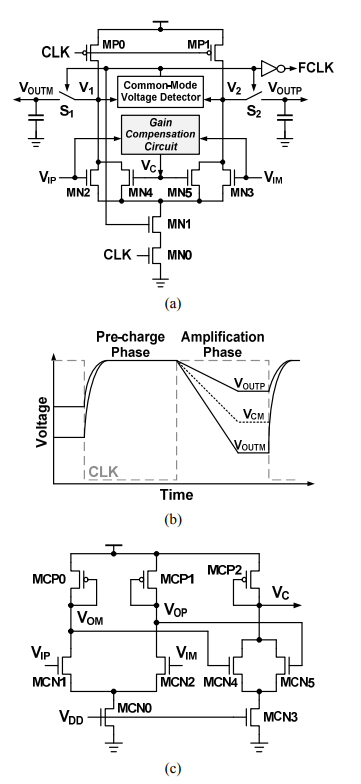On the other hand, the static voltage gain error of a residue amplifier of the pipelined ADC can be compensated using the digital error correction. However, the nonlinear variation of the voltage gain of the residue amplifier due to changes of the input voltage should be minimized or eliminated even if the digital error correction is used in the pipelined ADC. The nonlinear static error of the voltage gain is usually caused by the architecture of the dynamic amplifier using an open loop and the nonlinearity of the transistors used in the dynamic amplifier. It was reduced by supplying the control voltage V$_{C}$ to the two transistors MN4 and MN5 connected in parallel to the input transistors (3). Thus, the voltage gain of the dynamic amplifier (A$_{DIFF}$) is determined by Eq. (4),

##### (4)
\begin{aligned} \mathrm{A}_{\mathrm{DIFF}} =\left(\mathrm{V}_{\mathrm{OUTP}}-\mathrm{V}_{\mathrm{OUTM}}\right) /\left(\Delta \mathrm{V}_{\mathrm{N}}\right)=\left\{\mathrm{g}_{\mathrm{m} 2} /\left(\mathrm{I}_{\mathrm{D} 2}+\mathrm{I}_{\mathrm{D} 4}\right)\right\} \cdot\left(\mathrm{V}_{\mathrm{DD}}-\mathrm{V}_{\mathrm{CM}}\right) \end{aligned}

where I$_{D2}$ is the current at the operating point determined for the input common mode voltage of the input transistor MN2. However, the nonlinear variation of the voltage gain of the dynamic amplifier is not removed when the voltage of the V$_{C}$ is fixed. In this work, the gain compensation circuit shown in Fig. 6(c) is proposed to reduce the nonlinear variation of the voltage gain of the dynamic amplifier. The gain compensation circuit generates the control voltage V$_{C}$ that is controlled according to the voltages of the differential input signals V$_{IP}$ and V$_{IM}$ of the dynamic amplifier. The V$_{C}$ is derived by Eq. (5),

##### (5)
$\mathrm{V}_{\mathrm{C}}=\mathrm{V}_{\mathrm{DD}}-\left|\mathrm{V}_{\mathrm{th}_{-} \mathrm{MCP} 2}\right|-\left\{\beta \cdot \mathrm{V}_{\mathrm{CM}_{-} \mathrm{th}^{2}}+1 / 2 \cdot \beta \cdot \alpha^{2} \cdot\left(\mathrm{V}_{\mathrm{IP}}-\mathrm{V}_{\mathrm{IM}}\right)^{2}\right\}^{1 / 2}$

where ${\alpha}$ is the voltage gain of the pre-amplifier of the gain compensation circuit, V$_{CM\_th}$ is equal to (${\alpha}$ ·V$_{\mathrm{CM }}$${-}V_{\mathrm{th\_MN4}}), and {\beta} is defined to {{\mu}$$_{\mathrm{n}}$C$_{\mathrm{ox}}$(W/L)$_{\mathrm{MCN4}}$}/ {${\mu}$$_{\mathrm{p}}$C$_{\mathrm{ox}}$(W/L)$_{\mathrm{MCP2}}$}. When the two input voltages are equal to each other, V$_{C}$ has a maximum value. As the voltage difference between the two-input signals increases, the voltage of V$_{C}$ decreases as shown in Fig. 7(a), and the I$_{D4}$ decreases accordingly. This increases the voltage gain of the dynamic amplifier according to Eq. (4). Fig. 7(b) shows the simulation result that the voltage gain of the dynamic amplifier decreases as the V$_{C}$ increases. The linearity of the voltage gain of the dynamic amplifier is improved as the gain compensation circuit generates V$_{C}$ dependent on the voltage difference between the two input signals and supplies V$_{C}$ in parallel to the input signals of the dynamic amplifier. Fig. 7(c) shows the simulation results of the proposed dynamic amplifier. The variation of voltage gain according to the change of input voltage is reduced from ${\pm}$ 3.5% to ${\pm}$ 0.2% using the proposed gain compensation circuit when voltage gain is approximately 4.

Fig. 7. Simulation results of dynamic amplifier (a) control voltage according to differential input, (b) voltage gain curve according to control voltage, (c) voltage gain variation of proposed and conventional dynamic amplifiers.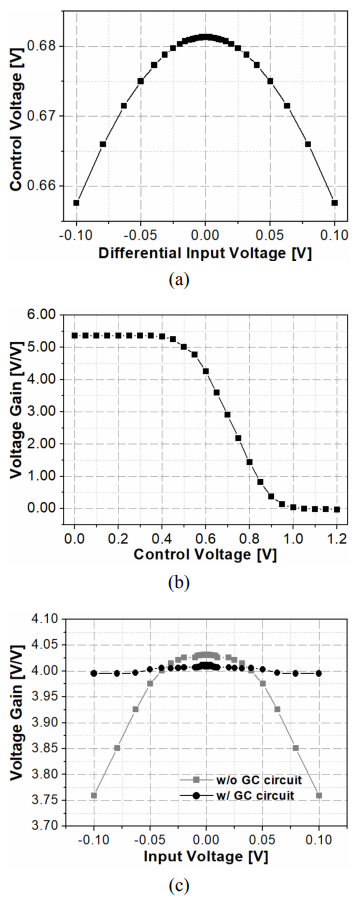Fig. 8. Proposed 6-bit fine SAR ADC (a) block diagram, (b) timing diagram.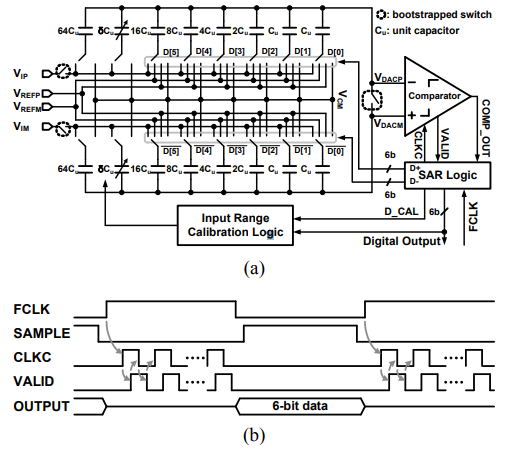### 3. 6-bit Fine SAR ADC with Input Range Calibration

The 6-bit fine SAR ADC has the architecture of an asynchronous SAR ADC such as the coarse SAR ADC and performs the input range calibration, as shown in Fig. 8(a). The binary weighted capacitors from 16·C$_{U}$ to 1·C$_{U}$ are used for the capacitor-based DAC with the VCM-based capacitor architecture used in the 6-bit SAR ADC. These capacitors are designed using MOM capacitors as in the 5-bit coarse SAR ADC. The 1·C$_{U}$ used in the 6-bit fine SAR ADC has a small capacitance of 2 fF to reduce the driving capability of the residue amplifier. The 6-bit fine SAR ADC is basically performed by the operation of an asynchronous SAR ADC. However, the 6-bit fine SAR ADC is operated in synchronization with FCLK, which is generated by the operation of the residue amplifier, instead of EX_CLK used for the sync signal of the 5-bit coarse SAR ADC, as shown in Fig. 8(b).

In the conventional pipelined ADC, the static voltage gain error of the residue amplifier is generally compensated in the digital error correction. However, because the voltage gain of the dynamic amplifier without feedback loop used as a residual amplifier is sensitive to the process, voltage, and temperature variations, the static voltage gain error of the dynamic amplifier may exceed the range that can be compensated by the digital error correction. Thus, the proposed SAR ADC reduces the effect of the static voltage gain error of the residue amplifier through the proposed input range calibration of the 6-bit fine SAR ADC. The proposed input range calibration is implemented by adding 64·C$_{U}$, ${\delta}$·C$_{U}$, and an input range calibration logic. The 64·C$_{U}$ reduces the voltage gain required in the dynamic amplifier from 16 to 4 (7). Furthermore, the ${\delta}$·C$_{U}$ is used to compensate the static gain error of the dynamic amplifier for the residue amplifier. The differential output voltage of the capacitor-based DAC used in the 6-bit fine SAR ADC is determined to Eq. (6). The digital code D is supplied to the capacitor-based DAC in only the proposed input range calibration mode.

##### (6)
$V_{D A C_{-} D I F F}=V_{I P}-V_{I M}+\frac{32}{64+\delta+32} \cdot V_{R} \cdot\left\{\frac{(-1)^{D}}{2^{0}}+\frac{(-1)^{D}}{2^{1}}+\cdots+\frac{(-1)^{D}}{2^{4}}+\frac{(-1)^{D}}{2^{5}}\right\}$

The ideal value of ${\delta}$ is 32 when the static gain error of the dynamic amplifier is not generated. Reflecting that the voltage gain required for the dynamic amplifier is reduced from 16 to 4, the differential voltage of the capacitor-based DAC, V$_{DAC\_DIFF}$, is determined to be 0 when the maximum voltages of V$_{IP}$ and V$_{IM}$ of the 6-bit fine SAR ADC are V$_{REFP}$/4 and V$_{REFM}$/4, respectively. The proposed input range calibration is performed by the operation of the proposed entire pipelined SAR ADC including the residue amplifier shown in Fig. 1. As the first process for the proposed input range calibration, the two input signals V$_{IP}$ and V$_{IM}$ of the proposed pipelined SAR ADC are connected to V$_{DD}$ and V$_{SS}$, respectively. In this condition, the ${\delta}$ is adjusted so that the V$_{DAC\_DIFF}$ is set to 0 after the D determined through the normal 10-bit data conversion is supplied to the capacitor-based DAC of the 6-bit fine SAR ADC. The optimum ${\delta}$ can be determined by detecting that D_CAL, the output of the comparator for the V$_{DAC\_DIFF}$ generated in the above case, is toggled between low and high. On the other hand, the last 1·C$_{U}$ of the capacitor-based DAC for the 6-bit SAR ADC is connected to V$_{CM}$ without being controlled by the D in the normal operation.

## III. CHIP IMPLEMENTATION AND MEASUREMENT RESULTS

The proposed 10-bit 100-MS/s pipelined SAR ADC was fabricated using a 65-nm 1-poly 8-metal CMOS process with a 1.2-V supply voltage, as shown in Fig. 9. Its active area is 410 ${\times}$ 425 ${\mu}$m$^{2}$. The total power consumption of the proposed ADC is 4.35mW at a sampling rate of 100-MS/s. In detail, the power consumption of the 5-bit coarse and 6-bit fine analog-to-digital converters is 1.961 mW and 1.89 mW, respectively. The power consumption of the dynamic amplifier with the gain compensation circuit consuming 17 ${\mu}$W is 422 ${\mu}$W. The digital error correction circuit, including the clock generator, consumes 347 ${\mu}$W of power.

Fig. 10 shows the measured static performances of DNL and INL. The input range calibration used in the 6-bit fine SAR ADC improved the performances of DNL and INL from +4.27/${-}$0.63 LSB and +3.46/${-}$3.21 LSB to +1.01/${-}$0.71 LSB and +1.52/${-}$1.53 LSB, respectively. Fig. 11(a) and (b) show the power spectrum of the ADC output measured at a sampling rate of 100-MS/s for a 2.4 V$_{\mathrm{PP}}$ differential sinusoidal input with frequency of 9.99 MHz. The input range calibration used in the 6-bit fine SAR ADC improved the effective number of bits (ENOBs) from 7.33 bits to 8.70 bits. Furthermore, the proposed pipelined SAR ADC maintains the ENOBs of 8.53 bits for the input signal with a frequency of 45.53 MHz close to the \textit{Nyquist} frequency, as shown in Fig. 11(c). The experimental results shown in Fig. 10 and 11 indicate that the proposed input range calibration used in the 6-bit fine SAR ADC improves the performance of the proposed pipelined SAR ADC by compensating for the variation in the voltage gain of the dynamic amplifier used as the residue amplifier.

Table 1. Performance summary and comparison

  TVLSI 2014  JSTS 2014  JSTS 2017  JSSC 2011  JSSC 2012 This work Technology 130 nm 110 nm 28 nm 65 nm 65 nm 65 nm Architecture Pipeline Hybrid Pipeline Pipelined SAR Pipelined SAR Pipelined SAR Pipelined SAR Supply [V] 1.2 1.1 1.0 1.1 1.1 1.2 Resolution [bit] 10 12 10 10 10 10 $\mathrm{f}_{\mathrm{s}}[\mathrm{MS} / \mathrm{s}]$ 75 100 160 40 160 100 ENOB [bit] 9.16(@DC) 8.85(@Nyquist) 9.71(@DC) 8.99(@Nyquist) 8.25(@DC) 8.2(@Nyquist) 8.86(@DC) 8.43(@Nyquist) 8.91(@DC) 8.44(@Nyquist) 8.70(@low freq.) 8.53(@Nyquist) DNL [LSB] +0.4/-0.53 +0.38/-0.37 +0.70/-0.71 - +0.46/-0.3 +1.01/-0.71 INL [LSB] +1.1/-0.82 +1.21/-1.21 +0.66/-0.70 - +1.3/-1.7 +1.52/-1.53 Power [mW] 32 25.3 5.6 1.21 2.72 4.35 Area [$\mathrm{mm}^{2}$] 0.65 1.34 0.23 0.06 0.21 0.17 FoM [fJ/c-s]  745.8(@DC) 924.6(@Nyquist) 302.0(@DC) 497.6(@Nyquist) 115.0(@DC) 119.0(@Nyquist) 65.1(@DC) 87.7(@Nyquist) 35.3(@DC) 48.9(@Nyquist) 104.6(@DC) 116.8(@Nyquist)

Fig. 10. Measured DNL/INL static performance (a) w/o input range calibration, (b) w/ input range calibration.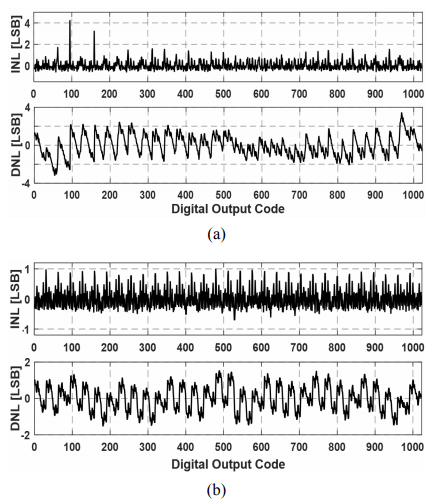Table 1 summarizes and compares the performances of ADCs with similar specifications to the proposed pipelined SAR ADC. The proposed ADC provides improved performance for the figure of merit (FoM) and area compared to ADCs with sampling rates of 100 MHz or higher using a single channel.

Fig. 11. Measured dynamic performance (a) w/o input range calibration for low frequency input signal, (b) w/ input range calibration for low frequency input signal, (c) w/ input range calibration for high frequency input signal.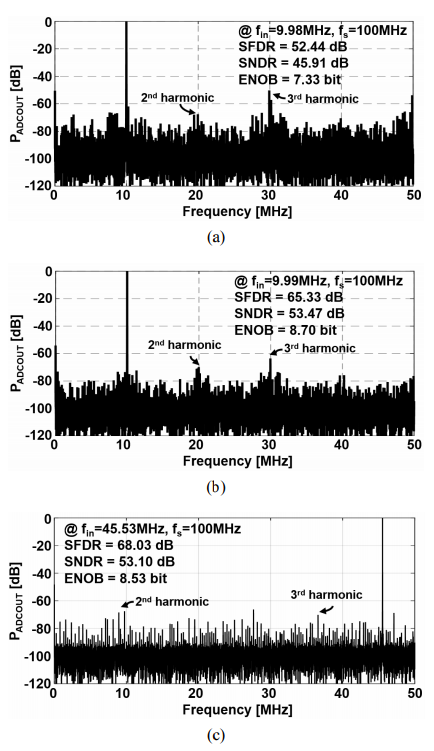## IV. CONCLUSIONS

The 10-bit 100-MS/s pipelined SAR ADC was implemented using a 65-nm 1-poly 8-metal CMOS process with a 1.2-V supply voltage. It consists of a 5-bit coarse SAR ADC with 1-bit redundancy, a dynamic amplifier for a residue amplifier, a 6-bit fine SAR ADC with input range calibration, and a digital error correction. The 1-bit redundancy generation, implemented using the capacitor-based DAC in the 5-bit SAR ADC for sub-ADC of the pipelined ADC was proposed to adopt the digital error correction using only adders. The dynamic amplifier with gain compensation circuit was used to improve the linearity of the residue amplifier while reducing its power consumption. The input range calibration used in the 6-bit fine SAR ADC improved the ENOBs from 7.33 bits to 8.70 bits at a sampling rate of 100 MS/s for a 2.4-V$_{\mathrm{PP}}$ differential sinusoidal input with frequency of 9.99 MHz.

### ACKNOWLEDGMENTS

This research was supported by the MOTIE (No. N0001883, HRD Program for Intelligent semiconductor Industry), the Basic Science Research Program (2016R1D1A3B03934487) through the National Research Foundation of Korea (NRF) funded by the Ministry of Education, Science and Technology, and in part by IDEC.

### REFERENCES

1
F. van der Goes , et. al. , 2014, A 1.5mW 68dB SNDR 80MS/s 2× Interleaved SAR-assisted pipelined ADC in 28nm CMOS, IEEE ISSCC, pp. 200-2012
V. Tripathi , et. al. , Sept. 2014, A 160 MS/s, 11.1 mW, single-channel pipelined SAR ADC with 68.3 dB SNDR, IEEE CICC3
J. Lin , et. al. , May 2011, A 15.5dB, Wide Signal Swing, Dynamic Amplifier Using a Common-Mode Voltage Detection Technique, IEEE ISCAS, pp. 21-244
H.-Y. Lee, , et. al. , Jun. 2018, A 10-bit 100-MS/s Pipelined SAR ADC with Input Range Calibration and Digital Error Correction, AWAD, pp. 256-2585
Y. Zhu , et. al. , June 2010, A 10-bit 100-MS/s reference-free SAR ADC in 90 nm CMOS, IEEE JSSC, pp. 1111-11216
S.-M. Park , et. al. , Jun 2016, A 10-bit 20-MS/s Asynchronous SAR ADC with Meta-stability Detector using Replica Comparators, IEICE transaction on Electronics, Vol. E99-C, No. 6, pp. 651-6547
Lee C. C., Flynn M. P., 2011, A SAR-Assisted Two-Stage Pipeline ADC, IEEE JSSC, Vol. 46, No. 4, pp. 859-8698
Woo J.-K., et.al. , Mar 2014, 1.2 V 10-bit 75 MS/s Pipelined ADC With Phase-Dependent Gain-Transition CDS, IEEE transactions on VLSI Systems, Vol. 22, No. 3, pp. 585-5929
Park J.-S., et.al. , Apr 2014, A 12b 100MS/s Three-Step Hybrid Pipeline ADC Based on Time-Interleaved SAR ADCs, IEIE JSTS, Vol. 14, No. 2, pp. 189-19710
An T.-J., et.al. , Oct 2017, A Two-channel 10b 160 MS/s 28 nm CMOS Asynchronous Pipelined-SAR ADC with Low Channel Mismatch, IEIE JSTS, Vol. 17, No. 5, pp. 636-64711
Furuta M., et.al. , Apr 2011, A 10-bit, 40-MS/s, 1.21 mW Pipelined SAR ADC Using Single-Ended 1.5-bit/cycle Conversion Technique, IEEE JSSC, Vol. 46, No. 6, pp. 1360-137012
Zhu \Y., et.al. , Sep 2012, A 50-fJ 10-b 160-MS/s Pipelined-SAR ADC Decoupled Flip-Around MDAC and Self-Embedded Offset Cancellation, IEEE JSSC, Vol. 47, No. 11, pp. 2614-2626## Author

Han-Yeol Lee was born in Gimcheon, Korea, on 1986.

He received the B.S., M.S., and Ph.D. degrees in the department of electronic engineering from Kumoh National Institute of Technology, Gumi, Korea, in 2012, 2014, and 2019, respectively.

In 2019, he joined the Driver IC Design Part, DB Hitek, Seoul, Korea, as a professional.

His current research interests are in the design of data converters.

Eunji Youn was born in Daejeon, Korea, on 1994.

She received the B.S. and M.S. degrees in the department of electronic engineering from Kumoh National Institute of Technology, Gumi, Korea, in 2017 and 2019, respectively.

In 2019, she joined the ICT Convergence Research Center, Kumoh National Institute of Technology, Gumi, Korea, as a researcher.

Her current research interests are in the design of data converters.

Young-Chan Jang was born in Daegu, Korea, on 1976.

He received the B.S. degree in the department of electronic engineering from Kyung-pook National University, Daegu, Korea, in 1999 and the M.S. and Ph.D. degrees in electronic engi-neering from Pohang University of Science and Technology (POSTECH), Pohang, Korea, in 2001 and 2005, respectively.

From 2005 to 2009, he was a Senior Engineer in the Memory Division, Samsung Electronics, Hwasung, Korea, working on high-speed interface circuit design and next-generation DRAM.

In 2009, he joined the School of Electronic Engineering, Kumoh National Institute of Technology, Gumi, Korea, as a Faculty Member, where he is currently Professor.

His current research area is high-performance mixed-mode circuit design for VLSI systems such as high-performance signaling, clock generation, and analog-to-digital conversion.